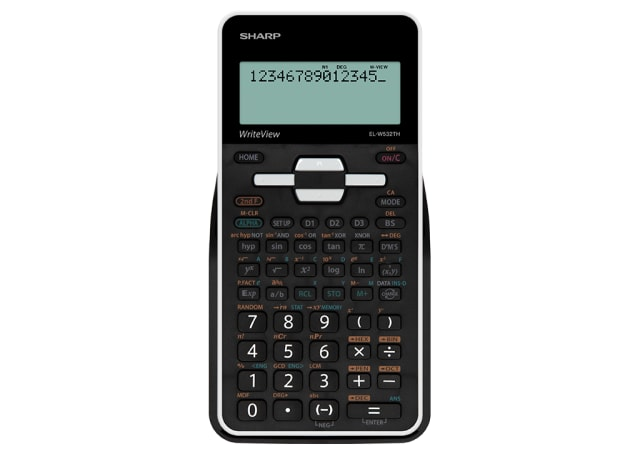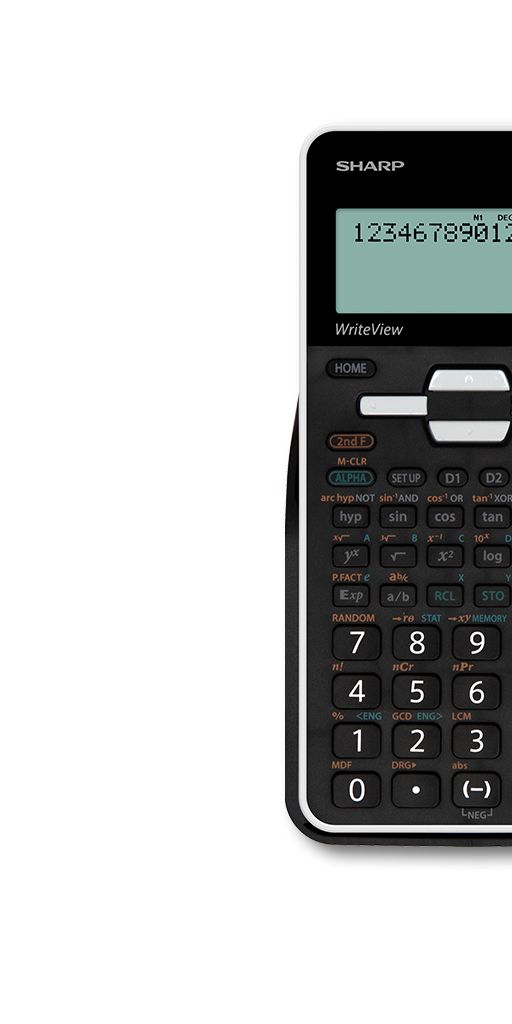ELW532THBWH

# WriteView Scientific Calculator

• WriteView Scientific Calculator
• Recommended High School Model
• Approved by the NSW Board Of Studies
• Successor to the ELW532XHBSL
• Large, high resolution, 4-line x 32 digit dot matrix LCD screen
• Up to 396 scientific and statistical functions
• Home key and √,π as 1st function arrangement
• Fraction calculations
• PRIME Factorization
• Drill Mode
POA## Scientific Calculator## LCD Display## Battery Operated## Protective Hard Cover• A scientific calculator designed for usage in science, engineering, and mathematics. Great for school/univeristy students or engineers.
• Four-line display showing equation entered on top line and answer on the bottom line allowing users to check both the equation and answer at the same time. User can amend the equation if needed.
• Powered by 1 x AAA battery. Battery is secured with child-safe lock.
• Recall previous equations, storing up to 142 characters. 9 values can be stored in calculator, with 8 temporary memories and 1 independent memory.
• Calculator is approved by the Board of Studies. Can be used in schools.
• Store up to 396 scientific and statistical functions in calculator.
• Hard cover to protect calculator from scratches and for easy storage. Great for students to store their calculators in their school bags.
• "A portion of the equation is automatically memorised as a constant ( function Plus, Minus, Division, Multiplication) , thus omitting redundant input. E.g. 2 x 5 = 10 pressing = will multiply by 5 again.
• This function is great for instances where you'll need to calculate the same equation with a different variable e.g. stocktake or estimating sales.
• The square root of a number is a value that when multiplied by itself equals the original number. Great for jobs like architects, carpenters and engineers.
• Provides mixed or specific Maths Drill exercises. Great for students looking for a quick test for themselves to ensure they are learning.
• Ensures symbols and numbers of an equation to be entered as they are written. Instead of wasting energy on difficult calculator operations, users are free to concentrate on mathematical concepts. E.g. You want to input 10 + 2 x sin30= You push the keys in the same order as the actual equation.
• Allows you to enter numbers as fractions.
• Allow equations to be entered without having to key the answer from previous equation repeatedly.
• To use the calculator for 1-2 variable statistic calculations. Great for students or engineers.
Calculator Types
Scientific Calculators
Display
4-line, 96 x 32 dot matrix LCD
Dimensions (WxHxD)
80 x 166 x 15 millimetre
Power Source
1 x AAA
Memory
9
Scientific & Stats function
396
Multi-line Playback
Yes
Chain Calculation
Yes
Fraction Key
Yes
Hard Cover
Yes, slide-on
Displayed Result
10 digit mantissa, 2 digit exponent
Internal Calculations
Mantissa of up to 14 digits